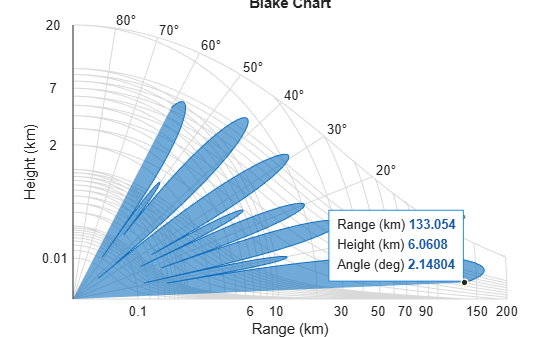# refractionexp

CRPL exponential reference atmosphere refraction exponent

Since R2021b

## Syntax

``rexp = refractionexp(Ns)``

## Description

example

````rexp = refractionexp(Ns)` computes the refraction exponent or decay constant of the CRPL Exponential Reference Atmosphere Model.```

## Examples

collapse all

Compute the refraction exponents for surface refractivities equal to `200` N-units, `313` N-units, and `450` N-units.

```srfrf = [200 313 450]; rexp = refractionexp(srfrf)```
```rexp = 1×3 0.1184 0.1439 0.2233 ```

Compute and plot the radar vertical coverage pattern for a sinc antenna pattern. Specify a frequency of 100 MHz, an antenna height of 10 meters, and a range of 100 km. Assume the surface is smooth, the antenna is not tilted, and the transmitted polarization is horizontal.

```frq = 100e6; anht = 10; rng = 100;```

To specify the effective Earth radius, assume a high-latitude atmosphere model and a winter-like seasonal profile. Use the `refractiveidx` function to compute the refractivity gradient in N-units per meter using the Earth's surface and an altitude of 1 km.

```alt1km = 1e3; [nidx,N] = refractiveidx([0 alt1km], ... LatitudeModel="High",Season="Winter"); RGrad = (nidx(2) - nidx(1))/alt1km; Re = effearthradius(RGrad);```

Compute the vertical coverage pattern using the effective Earth radius and the radar parameters.

```[vcpKm,vcpangles] = radarvcd(frq,rng,anht, ... EffectiveEarthRadius=Re);```

Use the refractivity at the surface in N-units to compute the refraction exponent.

```Ns = N(1); rexp = refractionexp(Ns)```
```rexp = 0.1440 ```

Plot the vertical coverage pattern in the form of a Blake chart.

```blakechart(vcpKm,vcpangles, ... SurfaceRefractivity=Ns,RefractionExponent=rexp)```## Input Arguments

collapse all

M-length refractivity at the surface in N-units, specified as a real scalar.

Example: `313`

Data Types: `double`

## Output Arguments

collapse all

Refraction exponent or decay constant in km–1, returned as nonnegative real scalar.

collapse all

### CRPL Exponential Reference Atmosphere Model

Atmospheric refraction evidences itself as a deviation in an electromagnetic ray from a straight line due to variation in air density as a function of height. The Central Radio Propagation Laboratory (CRPL) exponential reference atmosphere model treats refraction effects by assuming that the index of refraction n(h) and the refractivity N decay exponentially with height. The model defines

`$N=\left(n\left(h\right)-1\right)×{10}^{6}={N}_{\text{s}}{e}^{-{R}_{\text{exp}}h},$`

where Ns is the atmospheric refractivity value (in units of 10–6) at the surface of the earth, Rexp is the decay constant, and h is the height above the surface in kilometers. Thus

`$n\left(h\right)=1+\left({N}_{\text{s}}\text{\hspace{0.17em}}×{10}^{-6}\right){e}^{-{R}_{\text{exp}}h}.$`

The default value of Ns is 313 N-units and can be modified using the `SurfaceRefractivity` name-value argument in functions that accept it. The default value of Rexp is 0.143859 km–1 and can be modified using the `RefractionExponent` name-value argument in functions that accept it.

 Bean, B.R., and G.D. Thayer. "Central Radio Propagation Laboratory Exponential Reference Atmosphere." Journal of Research of the National Bureau of Standards, Section D: Radio Propagation 63D, no. 3 (November 1959): 315. https://doi.org/10.6028/jres.063D.031.

 Dutton, E. J., and G. D. Thayer. Techniques for Computing Refraction of Radio Waves in the Troposphere. National Bureau of Standards Technical Note 97. United States National Bureau of Standards, 1961, revised 1964.